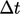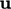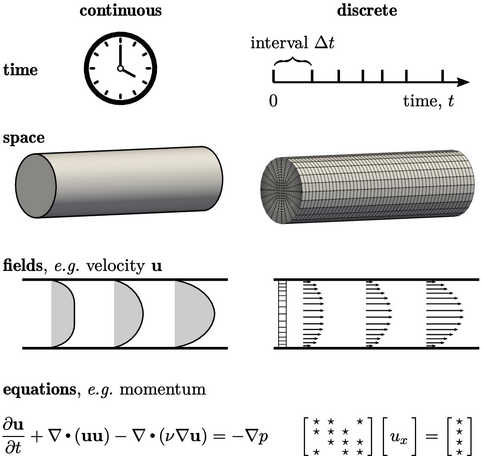## Chapter 3Numerical Method

“We were talking about the space between us all.”

The Beatles, Within You, Without You (1967).

Chapter 2 described:

• the main governing equations of ﬂuid dynamics, namely conservation laws of mass, momentum and energy;
• standard models for diﬀusion of momentum and heat, namely Newton’s law of viscosity and Fourier’s law of conduction;
• the ideal gas equation of state and speciﬁc heat capacity;
• tensor algebra used in the equations and the derivatives of time, divergence, gradient and Laplacian.

Sets of governing equations and models are combined to simulate diﬀerent ﬂow problems. Numerical methods are used in CFD to solve the equations since closed-form, analytical solutions only exist for very simple geometries and ﬂow regimes.

The numerical methods represent continuous physical entities by equivalent discrete entities, i.e.:

• time is split into intervals of duration;
• space becomes cells of a mesh — the solution domain;
• ﬁelds, e.g., become discrete values, e.g. one per cell;
• partial diﬀerential equations, e.g. for momentum, become a set of linear equations.

There are numerous discretisation methods to create the set of linear equations, but this book speciﬁcally examines the ﬁnite volume method.

The sets of equations can be solved using a variety of algorithms, with ﬁnite volume discretisation lending itself towards iterative methods.Notes on CFD: General Principles - Chapter 3 Numerical Method